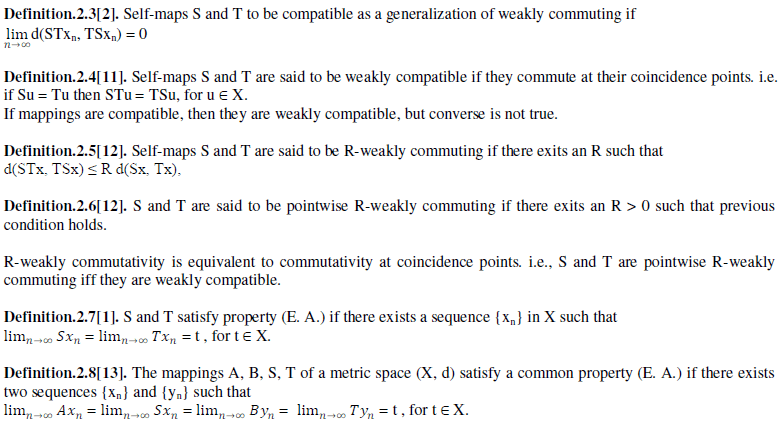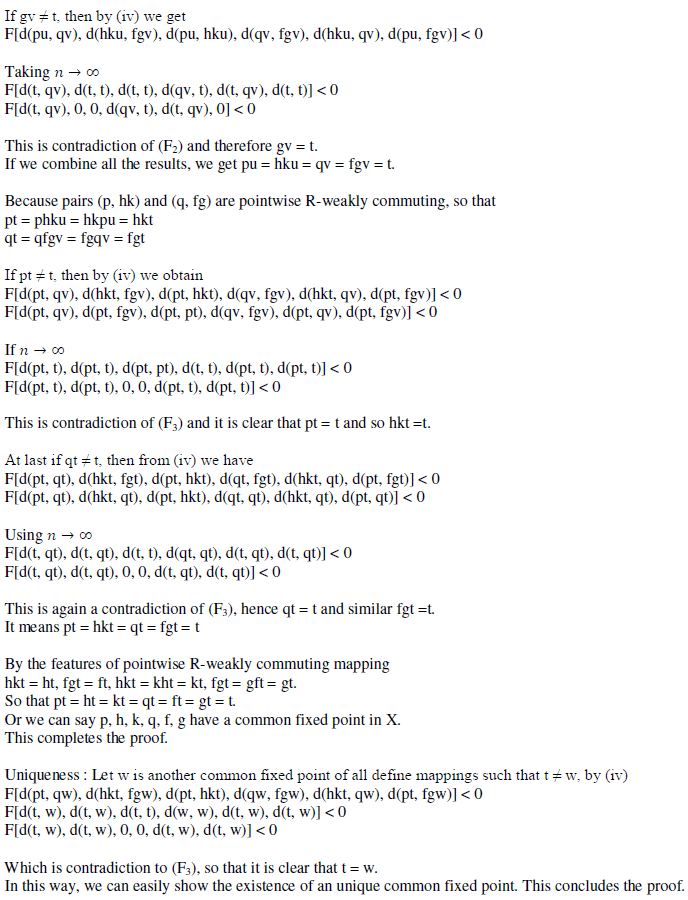ISSN ONLINE(2319-8753)PRINT(2347-6710)

Yakışıklı erkek tatil için bir beldeye gidiyor burada kendisine türk Porno güzel bir seksi kadın ayarlıyor Onunla beraber otel odasına gidiyorlar Otel odasına rokettube giren kadın ilk önce erkekle sohbet ederek işi yavaş halletmeye çalışıyor sex hikayeleri Kocası fabrikatör olan sarışın Rus hatun şehirden biraz uzak olan bir türk porno kasabaya son derece lüks bir villa yaptırıp yerleşiyor Kocasını işe gönderip mobil porno istediği erkeği eve atan Rus hatun son olarak fotoğraf çekimi yapmak üzere türk porno evine gelen genç adamı bahçede azdırıyor Güzel hatun zengin bir iş adamının porno indir dostu olmayı kabul ediyor Adamın kendisine aldığı yazlık evde sikiş kalmaya başlayan hatun bir süre sonra kendi erkek arkadaşlarını bir bir çağırarak onlarla porno izle yapıyor Son olarak çağırdığı arkadaşını kapıda üzerinde beyaz gömleğin açık sikiş düğmelerinden fışkıran dik memeleri ile karşılayıp içeri girer girmez sikiş dudaklarına yapışarak sevişiyor Evin her köşesine yayılan inleme seslerinin eşliğinde yorgun düşerek orgazm oluyor film izle
All submissions of the EM system will be redirected to Online Manuscript Submission System. Authors are requested to submit articles directly to Online Manuscript Submission System of respective journal.

# Common Fixed Point Theorem Governed by Implicit Relation and Property (E. A.)

 G. P. S. Rathore1, Bijendra Singh2, Kirty Chauhan3 College of Horticulture, Mandsaur, India S.S. in Mathematics, Vikram University, Ujjain, India Department of Mathematics, A T C, Indore, India Related article at Pubmed, Scholar Google

Visit for more related articles at International Journal of Innovative Research in Science, Engineering and Technology

## Abstract

In order to demonstrate the utility of implicit relation in metric space, we have added common fixed point theorem through this paper. It is a generalized work on pointwise R-weakly commuting and compatible mappings sharing the common property (E. A.). This work extends the results contained in available research work over compatible mappings and as a bi-product we obtain new theorem in metric spaces.

### Keywords

Compatible maps, pointwise R-weakly commuting mappings, property (E. A.), implicit relation

### INTRODUCTION

The theory of probabilistic metric spaces introduced by Menger , where a distribution function was used instead of non negative real number as value of the metric. Sehgal  derive the concept of contraction mapping theorems over there. Here it may be noted that the notion of compatible mapping is due to Jungck . This concept has been frequently uses to derive theorems on fixed points. Aamri and Moutawakil  introduced property (E. A.) and common property (E. A.), which is a successful and popular generalization of compatible and non compatible mappings in metric space. Their work extended by Imdad et al.  in field of semi metric spaces while Kubiaczyk and Sharma  developed it in Menger space under strict contractive conditions. The concept of weakly commuting mappings in PM spaces developed by Singh et al. . Kumar and Chugh  derived some theorems in metric spaces by using the idea of pointwise R-weakly commutativity. In present paper we utilize these concepts to prove our theorem for six mappings in PM space, which generalize known results of  and .

### PRELIMINARIES

A metric is like a function that satisfies the minimal properties we might except of a distance. We begin with some known definitions.
Definition.2.1. A metric d on a set X is a function d : X × X → [0, ∞) such that for all x, y ∈ X :
(i). d(x, y) ≥ 0 and d(x, y)= 0 iff x = y,
(ii). d(x, y) = d(y, x), (symmetry)
(iii). d(x, y) ≤ d(x, z) + d(z, y) (triangle inequality).
A metric space (X, d) is a set X with a metric d defined on X and has a notion of the distance d(x, y) between every pair of points x, y ∈ X.We can define many different metrics on the same set, but if the metric on X is clear from the context, we refer to X as a metric space and omit explicit mention of the metric d.
Definition.2.2. Self-maps S and T to be weakly commuting if d(STx, TSx) ≤ d(Sx, Tx), for ∀ x ∈ X### MAIN RESULT

To prove the fixed point theorem, we follow the idea of a class of implicit functions initiated by Popa , because it covers several contractive conditions rather than one.### CONCLUSION

In this paper through Theorem 3.2 we introduce the new concept of common fixed point in case of newly defined implicit relation.

### References

 Aamri, M. and Moutawakil, D., “Some new common fixed point theorems under strict contractive conditions”, J. Math. And anal. Appl., Vol.27, pp.181-188,2002.

 Jungck, G., “Compatible mappings and common fixed points”, Int. J. Math. Sci., Vol.9, pp.771-779, 1986.

 Menger, K., “Statistical metrics”, Proc. Nat. Acad. Sci., USA, Vol.28, pp.535 – 537, 1942.

 Kubiaczyk, I. and Sharma, S., “Some common fixed point theorems in Menger space under strict contractive conditions”, Southeast Asian bulletin of math., Vol.32, pp.117-124, 2008.

 Imdad, M., Ali, J. and Khan, L., “Coincidence and fixed points in symmetric spaces under strict contractions”, J. of Math. Ana. And App., Vol.320, pp.352-360, 2006.

 Sehgal, V. M. and Bharucha-Reid, A., “Fixed point of contraction mappings on PM space”, Math. Sys. Theory, Vol.6(2), pp.97-100, 1972.

 Aliouche, A., “Common fixed point theorems via an implicit relation and new properties”, Soochow J. Math., vol.33(42), pp.593-601, 2007.

 Popa, V., “Some fixed point theorems for compatible mappings satisfying an implicit relation”, Demonstratio math., Vol.32, pp.157-163, 1999.

 Kohli, J. K., Vashistha S. and Kumar D., “A common fixed point theorem for six mappings in PM spaces satisfying contractive type implicit relations”, I. J. Math. Ana., Vol.4(2), pp.63-74, 2010.

 Sessa, S., “On a weak commutativity condition of mappings in fixed point considerations”, Publ. Inst. Math., Vol.32:46, pp.149-153, 1982.

 Jungck, G., “Common fixed points for non continuous non self maps on non metric spaces”, Far east J. Math. Sci., Vol.4:2, pp.199-215, 1996.

 Pant, R. P., Common fixed points of non commuting mappings, J. Math. Anal. Appl., Vol.2, pp.436-440, 1994.

 Liu, W., Wu, J. and Li, Z., “Common fixed points of single valued and multi valued maps”, J. Math. Math. Sci., Vol.19, pp.3045-3055, 2005.

 Singh, S. L., Pant B. D. and Talwar R., “Fixed points of weakly commuting mappings on menger spaces”, Jnanabha, Vol.23, pp.115-122, 1993.

 Kumar, S. and Chugh, R., “Common fixed point theorems using minimal commutativity and reciprocal continuity conditions in metric spaces”, Sci. Math. Japan, Vol.56, pp.269-275, 2002.